Exam 1 Review Problems

1) Suppose that the observational units in a study are the patients arriving at an emergency room in a given day. For each of the following, indicate whether it can legitimately be considered a variable or not. If it is a variable, classify it as categorical (and if it is binary) or quantitative. If it is not a variable, explain why not.

a. Blood type

b. Waiting time

c. Mode of arrival (ambulance, personal car, on foot, other)

d. Whether or not men have to wait longer than women

e. Number of patients who arrive before noon

f. Whether or not the patient is insured

g. Number of stitches required

h. Whether or not stitches are required

i. Which patients require stitches

j. Number of patients who are insured

k. Assigned room number

2) When a tennis racquet is spun, is it equally likely to land with its label facing up or down? (This technique is often used to decide who should serve first.) Or does the spinning process favor one outcome more than the other? A statistics professor once investigated this question by spinning his tennis racquet many times. For each spin he recorded whether the racquet landed with the label up or down.

(a) Describe (in words) the relevant parameter whose value is being investigated with this study.

(b) Write the appropriate null and alternative hypotheses (in symbols).

He spun his racquet 100 times, finding that it landed with the label up in 46 of those spins.

(c) Would you consider these 100 spins to be a sample from a random process or a random sample from a population? Explain briefly.

(d) If you were to carry out a simulation analysis to decide whether this result constitutes strong evidence that his racquet spinning process is not equally likely to land with its label facing up or down, what would you enter in these boxes?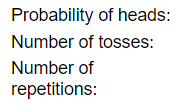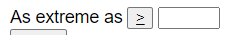(e) Based on the output below, what is the simulation-based p-value?(f) What are the parameters of the binomial distribution: n = and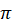=  and we found P(X   ?   )?

(g) Check whether the normal approximation (Central Limit Theorem) is valid here.

(h) Describe what the CLT says about the (approximate) sampling distribution of the sample proportion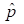, assuming that the null hypothesis is true. Be sure to describe each of shape, mean, and standard deviation, and to include a rough sketch (but well labeled) of the distribution.

(i) If the null hypothesis is true, and we repeatedly take samples of 100 spins from this process and calculate the proportion landing with label up, 95% of sample proportions should fall between what two values?

(j) Calculate (by hand) and interpret the test statistic by finding the z-score for the observed sample proportion.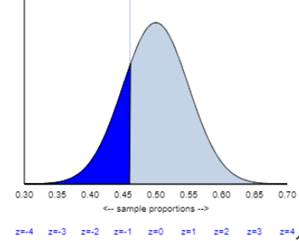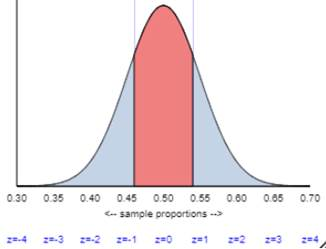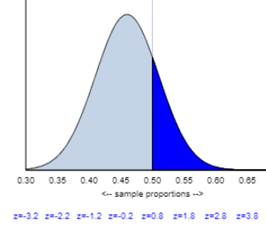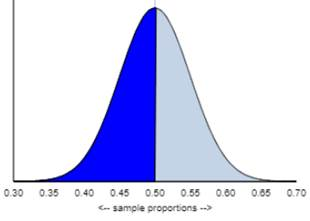(l) What test decision would you make at the .05 significance level?

(m) Do the validity conditions for the (Wald) normal-based confidence interval (one sample z-interval) hold here?

(n) Produce and interpret a 95% confidence interval for the parameter, using the Wald procedure (one sample z-interval) if the conditions are met but using the Plus Four procedure if they are not met.

(p) Is the confidence interval consistent with the test decision? Explain.

(q) Summarize your conclusion about the original question that motivated this study.

(r) Summarize how your calculations and conclusions would change if you instead examined the 54 spins that landed label down.

(s) Use the normal approximation to determine how large the sample size n needs to be in order for the 95% confidence interval to have margin-of-error < .08.

3) Findings at James Madison University indicate that 21% of students eat breakfast 6 or 7 times a week.  A similar question was asked of a sample of 159 Cal Poly students. Of the 97 who responded, 35 reported eating breakfast 6 or 7 times a week.  Is this convincing evidence that Cal Poly students have healthier breakfast habits (i.e., more likely to eat breakfast) than James Madison students?  More specifically, are you convinced that more than 21% of all Cal Poly students eat breakfast 6 or 7 times weekly?

(a) Define the population of interest and the sample being considered.

(b) Define the parameter and the statistic for this study.

(c) Is the population size large compared to the sample size to allow us to use the binomial model to this sampling process?

(d) Estimate a p-value for addressing this question. Clearly explain how you are doing so and report this p-value.

(e) What conclusion would you draw from this p-value?

(f) Provide an interpretation of this p-value as if to someone not taking a statistics class.

(g) If you took another random sample of 159 Cal Poly students, which of your answers to part b would change?

(h) What are your thoughts about the fact that only 97 out of the original random sample of 159 responded?

(i) Suppose you plan to conduct a new study with a simple random sample of 1,590 Cal Poly students. Explain how you could obtain this sample.

(j) Would this new sample size address the issue you identified in part h?

(k) How would you expect this p-value in part d to change if of the 1,590 Cal Poly students you sample 36% reported eating breakfast 6 or 7 times a week (larger, smaller, or about the same)? Explain (without finding a new p-value!).